# Digital Comparison Logic Using Multisim PLD and Digilent Teaching Hardware

Publish Date: Jun 15, 2018 | 1 Ratings | 5.00 out of 5 | Print | Submit your review

## Overview

Taking a hands-on approach to learning digital logic can be difficult without the need for students to learn complex hardware descriptive languages (eg. VHDL). Multisim Programmable Logic Diagram (PLD) along with support for leading Digilent teaching hardware allows students to put the fundamentals of digital theory into practice. The PLD schematic allows educators and students to create graphical logic diagrams like those found in textbooks and deploy these to Digilent educational boards. In this set of tutorials we demonstrate how digital logic theory can be taught using educational hardware to provide a hands-on approach to learning.

View the complete Teaching Digital Logic Fundamentals tutorial series

### 1. Introduction

Digital comparators are common combinational logic circuits used in CPUs and microcontrollers. The basic function of a digital comparator is to compare two binary quantities and generate a 1 or a 0 at the output depending on whether they are equal or not. In this tutorial we will design a parallel binary comparator circuit that compares a 4-bit binary number A to a 4-bit binary number B. If the binary quantities are equal, the output will generate a HIGH level.

The Multisim PLD Schematic will be used to capture and simulate the comparator circuit and, for a hands-on approach to learning, the PLD Schematic will be exported to a Digilent Nexys 4 FPGA board so that students can interact with real hardware.

Whilst this tutorial and the accompanying example were produced using the Digilent Nexys 4 the same could be achieved on other Digilent boards. More details on installing and deploying to the range of Digilent boards can be found here:

Getting Started with Digilent Boards in Multisim

### 2. Design

Based on the logic gates theory we know that the exclusive-NOR (XNOR) gate can be used as a basic comparator because its output is 1 only when the two inputs are equal. To demonstrate this we can use the Logic converter instrument in Multisim to obtain the truth table for the XNOR gate.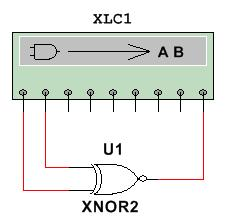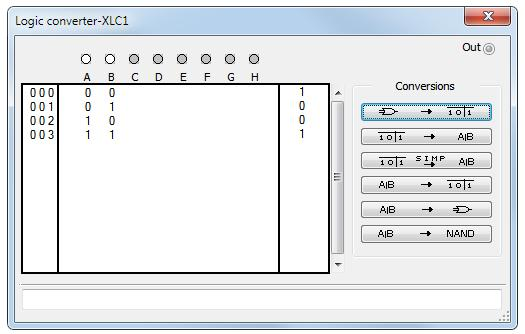In order to compare binary numbers with two bits each, we can use an additional XNOR gate and an AND gate, as shown below: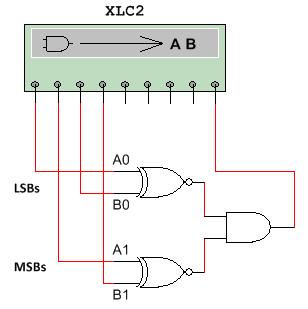As you can see, one of the XNOR gates compares A0 and B0, which are the least significant bits (LSBs). The most significant bits (MSBs), A1 and B1, are compared by the bottom XNOR gate. Finally, the AND gate evaluates the outputs of the XNOR gates to determine equality. When the two inputs bits for each XNOR gate are equal, their outputs are 1 and therefore the output of the AND gate is 1, this indicates equality.

In the next figure you can see the truth table for the 2-bit comparator circuit.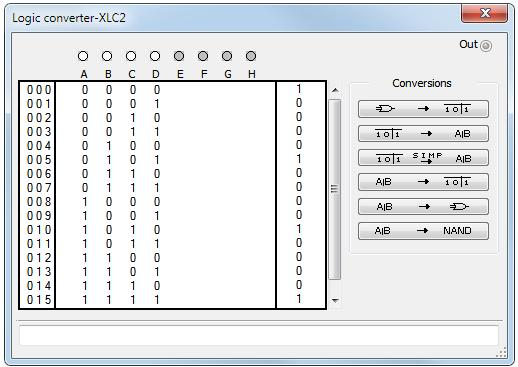Following the same approach, we can build a 4-bit comparator using four XNOR gates and one AND gate with four inputs, as shown below: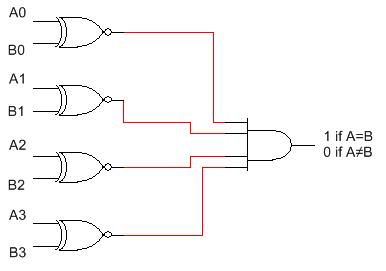### 3. Simulation

In this section we use the Multisim PLD Schematic to simulate the 4-bit parallel binary comparator. We will create a top level schematic with all the necessary components (for instance: switches) to simulate the circuit and also a PLD subcircuit with all the digital logic.

1. Open Multisim and select File » New » Blank to create new design.
2. Select Place » New PLD subcircuit. This will open the New PLD Design window.
3. Select the Digilent board you will be using from the drop-down menu. For this example we will use the Digilent Nexys 4 board, as shown below. Click Next.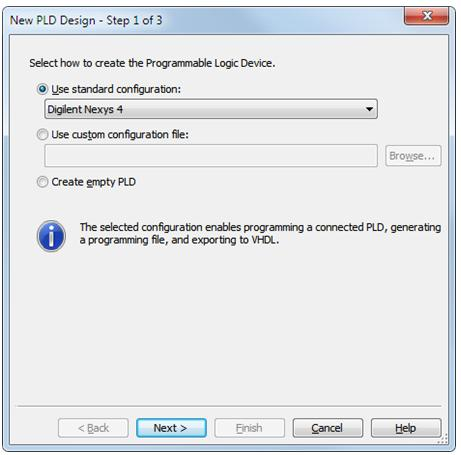1. Provide a name for the PLD subcircuit and click Next.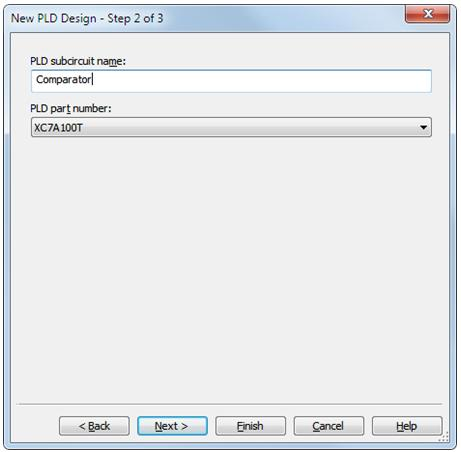1. Select the connectors that you will be using on the PLD schematic. For this example we use connectors SW0 to SW7 which correspond to the slide switches in the physical board. Bits A0 to A3 are represented by SW0 to SW3, and bits B0 to B3 by SW4 to SW7, respectively. Also, select LED0 for the output of the circuit.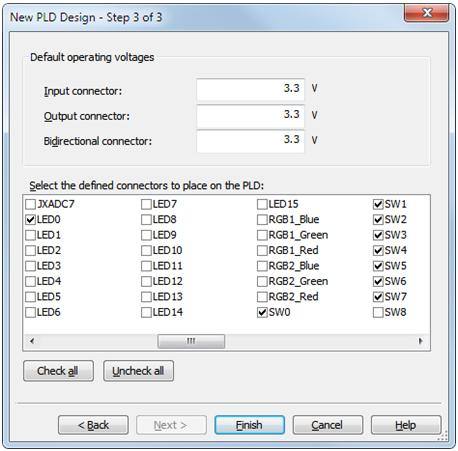1. Click Finish and place the PLD subcircuit on the workspace.
2. Select Place»Component and place the following components on the workspace:

 Component Group Family VCC Sources POWER_SOURCES DSWPK_8 Basic SWITCH PROBE_ORANGE Indicators PROBE

1. Wire the circuit as shown in the next figure.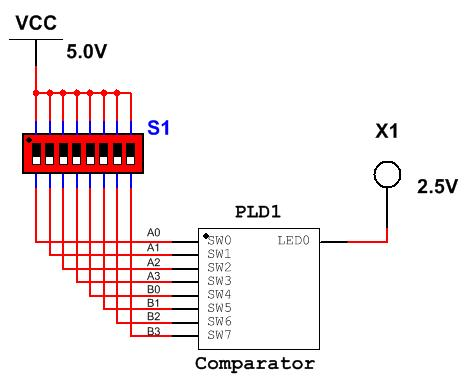The switch pack (S1) and the probe (X1) will be used to simulate the slides switches (SW0 to SW7) and LED0 of the Nexys 4 board.

1. Double-click the PLD subcircuit and select Open subsheet. The PLD schematic opens with all inputs (SW0 to SW7) on the left-hand side and the output (LED0) on the right-hand side. This is the place where we are going to define the internal logic behavior of the comparator circuit.
1.  Select Place » Component and place the following components on the workspace:

 Component Group Family AND4 PLD Logic LOGIC_GATES XNOR2 (4 gates) PLD Logic LOGIC_GATES

1. Wire the circuit as shown in the next figure.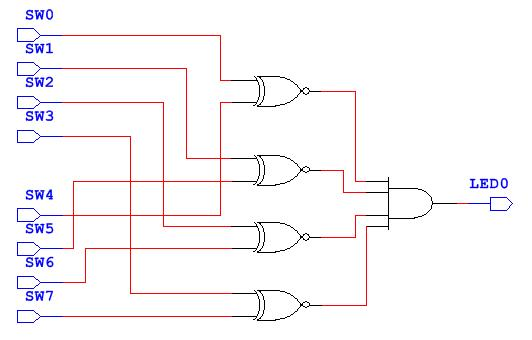1. Return to the top level circuit and run the simulation to verify the operation of the parallel binary comparator. Use the switch pack to enter different values of binary numbers. The probe will illuminate when the inputs are exactly equal.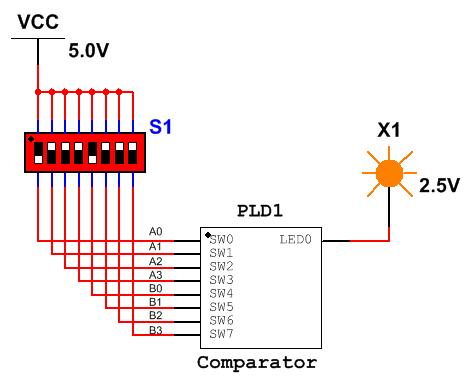### 4. Deployment

Now we are ready to deploy the PLD schematic to the Digilent Nexys 4 FPGA board. Connect the board to your computer and follow the next steps.

1. Double-click the PLD subcircuit and select Open subsheet.
2. Select Transfer»Export to PLD.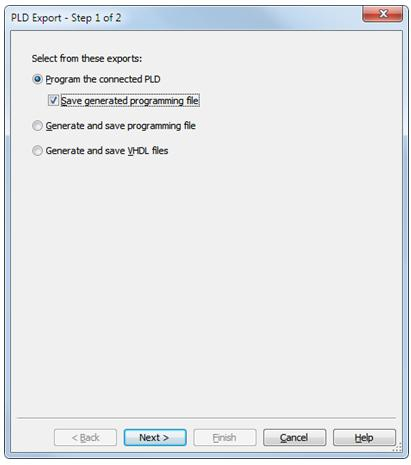1. Select Program the connected PLD and click Next.
2. Click the Refresh button to make sure the Digilent board has been detected.
3. Click Finish. Multisim will start the programming process.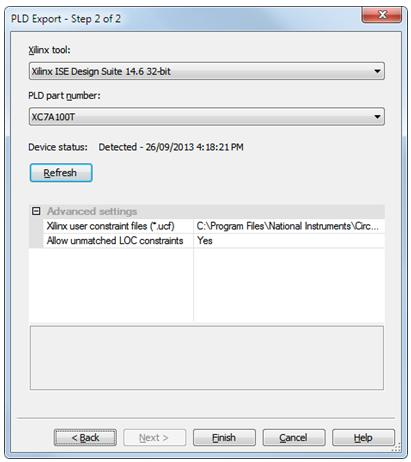After the PLD schematic have been successfully deployed to the Digilent board, experiment with the switches SW0 to SW7 to confirm the operation of the parallel binary comparator; observe the result in LED0.

All the files for this tutorial can be found in the attached file. This tutorial provides an example of how students can design, simulate and deploy digital logic with Multisim and Digilent FPGA boards

### Ratings

Rate this document